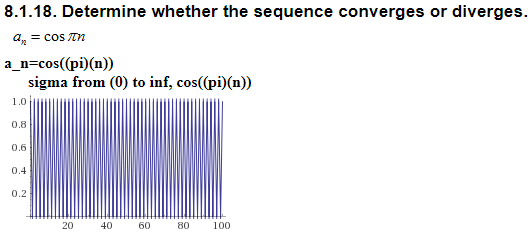# 8.1.18. Determine whether the sequence converges or diverges. a cos tn a-n=cos(pi)(n)) sigma from (0) to inf, cos(pi)(n)) 1.0 0.8 0.6 0.4 0.2 20 40 60 80 100

Question

### 8.1.18. Determine whether the sequence converges or diverges.

a_n=cos((pi)(n))

sigma from (0) to inf, cos((pi)(n))help_outlineImage Transcriptionclose8.1.18. Determine whether the sequence converges or diverges. a cos tn a-n=cos(pi)(n)) sigma from (0) to inf, cos(pi)(n)) 1.0 0.8 0.6 0.4 0.2 20 40 60 80 100 fullscreen

1 Rating

### Want to see this answer and more?

Experts are waiting 24/7 to provide step-by-step solutions in as fast as 30 minutes!*

*Response times vary by subject and question complexity. Median response time is 34 minutes and may be longer for new subjects.
Tagged in
MathCalculus

### Limits